﻿ Derivation of Continuous Linear Multistep Methods Using Hermite Polynomials as Basis Functions

### Derivation of Continuous Linear Multistep Methods Using Hermite Polynomials as Basis Functions

T. Aboiyar., T. Luga., B.V. Iyorter

American Journal of Applied Mathematics and Statistics

## Derivation of Continuous Linear Multistep Methods Using Hermite Polynomials as Basis Functions

T. Aboiyar.1, T. Luga.1, B.V. Iyorter2,1Department of Mathematics/Statistics/Computer Science, University of Agriculture, Makurdi, Nigeria

2Department of Mathematics and Computer Science, University of Mkar, Mkar, Nigeria

### Abstract

This paper concerns the derivation of continuous linear multistep methods for solving first-order initial value problems (IVPs) of ordinary differential equations (ODEs) with step number k=3 using Hermite polynomials as basis functions. Adams-Bashforth, Adams-Moulton and optimal order methods are derived through collocation and interpolation technique. The derived methods are applied to solve two first order initial value problems of ordinary differential equations. The result obtained by the optimal order method compared favourably with those of the standard existing methods of Adams-Bashforth and Adams-Moulton.

• T. Aboiyar., T. Luga., B.V. Iyorter. Derivation of Continuous Linear Multistep Methods Using Hermite Polynomials as Basis Functions. American Journal of Applied Mathematics and Statistics. Vol. 3, No. 6, 2015, pp 220-225. http://pubs.sciepub.com/ajams/3/6/2
• Aboiyar., T., T. Luga., and B.V. Iyorter. "Derivation of Continuous Linear Multistep Methods Using Hermite Polynomials as Basis Functions." American Journal of Applied Mathematics and Statistics 3.6 (2015): 220-225.
• Aboiyar., T. , Luga., T. , & Iyorter, B. (2015). Derivation of Continuous Linear Multistep Methods Using Hermite Polynomials as Basis Functions. American Journal of Applied Mathematics and Statistics, 3(6), 220-225.
• Aboiyar., T., T. Luga., and B.V. Iyorter. "Derivation of Continuous Linear Multistep Methods Using Hermite Polynomials as Basis Functions." American Journal of Applied Mathematics and Statistics 3, no. 6 (2015): 220-225.

 Import into BibTeX Import into EndNote Import into RefMan Import into RefWorks

### 1. Introduction

Linear multistep methods (LMMs) are very popular for solving initial value problems (IVPs) of ordinary differential equations (ODEs). They are also applied to solve higher order ODEs. LMMs are not self-starting hence, need starting values from single-step methods like Euler’s method and Runge-Kutta family of methods.

The general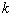-step LMM is as given in Lambert (1973)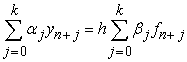(1)

where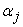and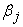are uniquely determined and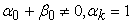.

The LMM in Equation (1) generates discrete schemes which are used to solve first-order ODEs. Other researchers have introduced the continuous LMM using the continuous collocation and interpolation approach leading to the development of the continuous LMMs of the form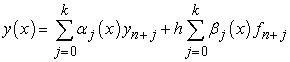(2)

whereandare expressed as continuous functions of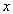and are at least differentiable once .

According to Okunuga and Ehigie , the existing methods of deriving the LMMs in discrete form include the interpolation approach, numerical integration, Taylor series expansion and through the determination of the order of LMM. Continuous collocation and interpolation technique is now widely used for the derivation of LMMs, block methods and hybrid methods.

Several continuous LMMs have been derived using different techniques and approaches: Alabi  derived continuous solvers of IVPs using Chebyshev polynomial in a multistep collocation technique; Okunuga and Ehigie  derived two-step continuous and discrete LMMs using power series as basis function; Mohammed  derived a linear multistep method with continuous coefficients and used it to obtain multiple finite difference methods which were directly applied to solve first-order ODEs; Odekunle et al  developed a continuous linear multistep method using interpolation and collocation for the solution of first-order ODE with constant stepsize; Adesanya et al [2, 3] considered the method of collocation of the differential system and interpolation of the approximate solution to generate a continuous LMM, which is solved for the independent solution to yield a continuous block method; James et al [11, 12] proposed a continuous block method for the solution of second order IVPs with constant stepsize, the method was developed by interpolation and collocation of power series approximate solution; Anake  developed a new class of continuous implicit hybrid one-step methods capable of solving IVPs of general second order ODEs using the collocation and interpolation techniques of the power series approximate solution; James et al [11, 12] adopted the method of collocation and interpolation of power series approximate solution to generate a continuous LMM; Ehigie et al  proposed a two-step continuous multistep method of hybrid type for the direct integration of second order ODEs in a multistep collocation technique; Akinfenwa et al  developed a four step continuous block hybrid method with four non-step points for the direct solution of first-order IVPs; Adesanya et al [2, 3] adopted the method of collocation of the differential system and interpolation of the approximate solution at grid and off grid points to yield a continuous LMM with constant stepsize and James et al [11, 12] developed a continuous block method using the approach of collocation of the differential system and interpolation of the power series approximate solution.

The introduction of continuous collocation schemes is of great importance as better global error can be estimated and approximations can be equally obtained. Also, the gap between the discrete collocation methods and the conventional multistep methods is bridged. In this study, we will develop continuous multistep collocation methods for the solution of first-order IVPs of ODEs using the probabilists’ Hermite polynomials as the basis function. The corresponding discrete schemes shall also be obtained.

### 2. Methods

In Awoyemi  and Onumanyi et al , some continuous LMM of the type in Equation (2) were developed using the collocation function of the form: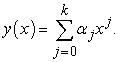(3)

Awoyemi et al  proposed a similar function of the type in Equation (3)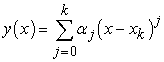(4)

to develop LMM for the solution of third-order IVPs. Adeniyi and Alabi  used Chebyshev polynomial function of the form: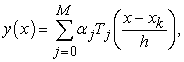where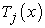are some Chebyshev function to develop continuous LMM.

In this paper, we propose the Probabilists’ Hermite polynomial of the form :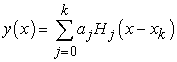where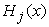are probabilists’ Hermite polynomials generated by the formula: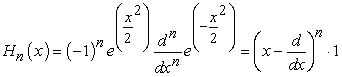and whose recursive relation is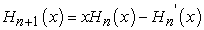to develop continuous LMMs for the solution of firs-order IVPs of ODEs of the form: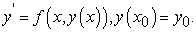(5)

The first six probabilists’ Hermite polynomials are: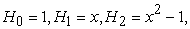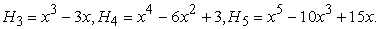2.1. Derivation of the Linear Multistep Methods

We wish to approximate the exact solution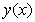to the IVP in Equation (5) by a polynomial of degree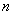of the form: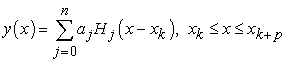(6)

which satisfies the equations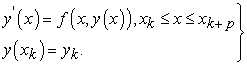(7)

To derive the three-step Adams-Bashforth method, we set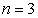in Equation (6), yielding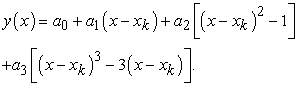(8)

Differentiating once gives the equation: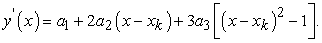(9)

Interpolating Equation (8) at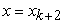and collocating Equation (9) at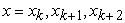yields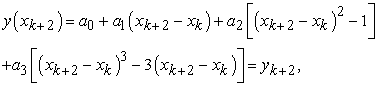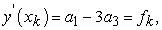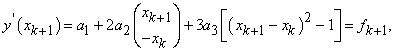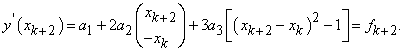In matrix form, we have: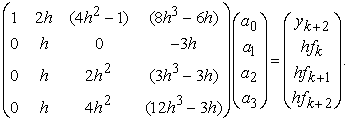Solving the system of equations by Gaussian elimination we obtain: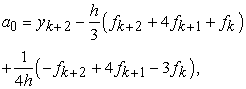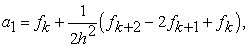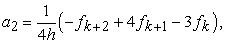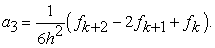Substituting for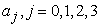in Equation (8) yields the continuous method: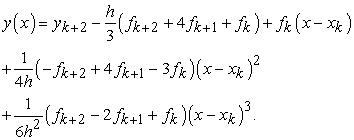(10)

Evaluating Equation (10) at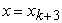, we obtain the discrete form as: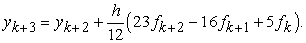(11)

To derive the three-step Adams-Moulton method, we let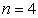in Equation (6) and differentiate the obtained function once. This gives: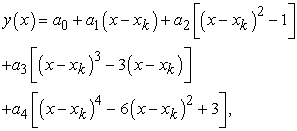(12)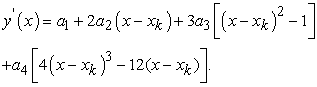(13)

Interpolating Equation (12) atand collocating Equation (13) at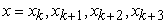gives to the equations: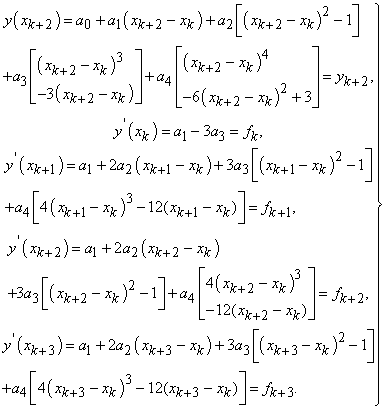(14)

The equations can be written in matrix form: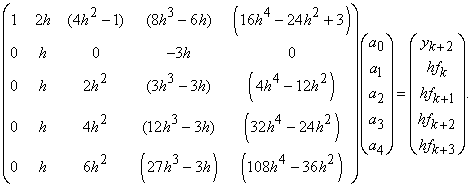Solving the system of equations by Gaussian elimination, we have that: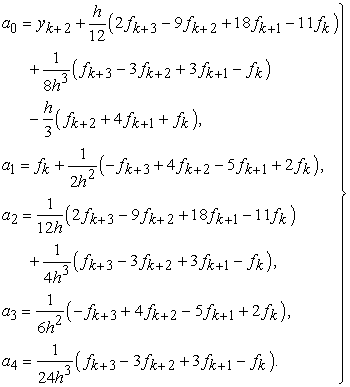(15)

Substituting for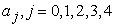in Equation (12) yields the continuous method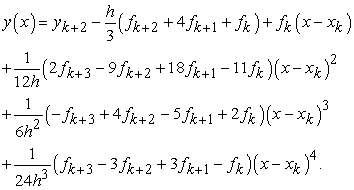(16)

Evaluating Equation (16) at, we obtain the discrete scheme: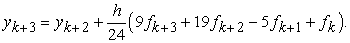(17)

The Optimal Order Method

The optimal order scheme is an implicit multistep method similar to the Adams-Moulton method. To derive the three-step optimal order method, we shall consider the system of equations in Equation (14) except for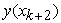. Interpolating Equation (12) at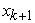we have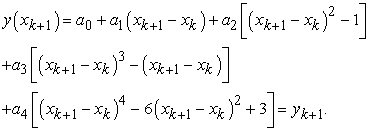The corresponding matrix of the equations is: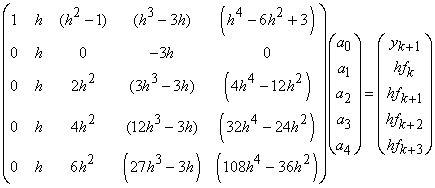Solving the system of equations give the same result as in Equation (15) above except for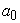. Thus, we haveto be: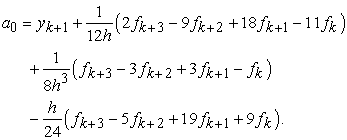Substituting forin Equation (12) yields the continuous three-step optimal order method: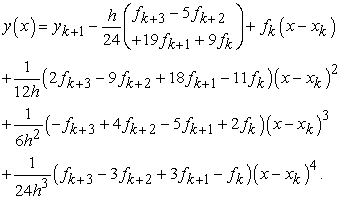(18)

Evaluating Equation (18) at, we obtain the discrete form: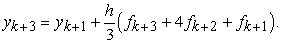(19)

### 3. Numerical Examples

In this section, we will apply the derived three-step methods of Adams-Barshforth, Adams-Moulton and the proposed optimal order to solve two IVPs of ODEs. Errors associated with the methods will also be obtained. The four-stage Runge-Kutta method is used to obtain the starting values, and the four-stage Adams-Bashforth method is used as a predictor to the implicit schemes. The result and errors obtained are tabulated for clarity.

Example 1

Consider the IVP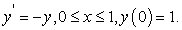Exact Solution: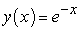.

Example 2

Consider the IVP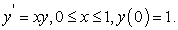Exact Solution: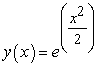.

The error is defined as: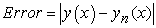whereis the exact solution and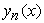is the approximate solution.

### 4. Discussion

Three continuous and discrete LMMs are derived through the technique of collocation and interpolation using the probabilists’ Hermite polynomials as basis functions. The result has shown that continuous and discrete LMMs can be derived using any polynomial function and approach. Table 1-Table 4 presents the results by the three derived methods: Equation (11), (17) and (19). Specifically, Table 2 and Table 4 give the errors by these methods. From the results obtained, the Adams-Moulton method produced better results than the Adams-Bashforth method but the proposed optimal order method is the most accurate.

### 5. Conclusion

The approach and basis functions applied in deriving the LMMs in this paper are different from those of some other researchers, though, the obtained LMMs (continuous and discrete) are same. Also, the proposed optimal order scheme has shown superiority over the standard existing methods of Adams-Bashforth and Adams-Moulton of the same step number, in terms of accuracy.

### References

  Adeniyi, R. B. and Alabi, M. O. (2006). Derivation of Continuous Multistep Methods Using Chebyshev polynomial Basis Function. Abacus, Vol 33 pp 351-361.In article  Adesanya, A. O., Momoh, A. A., Alkali, M. A. and Tahir, A. (2012). Five Steps Block Method for the Solution of Forth Order ODEs. International Journal of Engineering Research and Applications, Vol. 2(5) pp 991-998.In article  Adesanya, A. O., Odekunle, M. R., Remilekun, M. and James, A. A. (2012). Order Seven Continuous Hybrid Method for the Solution of First Order ODEs. Canadian Journal on Science and Engineering Mathematics, Vol. 3(4).In article  Akinfewa, O. A., Yao, N. M. and Jator, S. N. (2011). A Linear Multistep Hybrid Method with Continuous Coefficient for Solving Stiff ODE. Journal of Modern Mathematics and Statistics, Vol. 5(2) pp 47-53.In article View Article  Alabi, M. O. (2008). A Continuous Formulation of Initial Value Solvers with Chebyshev Basis Function in a Multistep Collocation Technique. PhD Thesis. Department of Mathematics, University of Ilorin. 136pp.In article  Anake, T. A. (2011). Continuous Implicit Hybrid One-Step Methods for the Solution of Initial Value Problems of General Second Order ODEs. PhD Thesis. School of Postgraduate Studies, Covenant University, Ota, Nigeria. 170pp.In article  Awoyemi, D. O (1999). A Class of Continuous Methods for the Solution of General Second Order Ordinary Differential Equations. International Journal of Computational Mathematics, Vol 72 pp 29-37.In article View Article  Awoyemi, D. O., Kayode, J. S. and Adoghe, L. O. (2014). A Four-Point Fully Implicit Method for the Numerical Integration of Third-Order Ordinary Differential Equations. International Journal of Physical Sciences, Vol 9(1) pp 7-12.In article View Article  Ehigie, J. O., Okunuga, S. A., Sofoluwe, A. B. and Akanbi, M. A. (2010). On Generalized Two-Step Continuous Linear Multistep Method of Hybrid Type for the Integration of Second Order ODEs. Archieves of Applied Science and Research, Vol. 2(6) pp 362-372.In article  James, A. A., Adesanya, A. O. and Sunday, J. (2013). Continuous Block Method for the Solution of Second Order Initial Value Problems of ODEs. International Journal of Pure and Applied Mathematics, Vol. 83(3) pp 405-416.In article View Article  James, A. A., Adesanya, A. O., Odekunle, M. R. and Yakubu, D. G. (2013). Constant Order Predictor Corrector Method for the Solution of Medeled Problems of First Order Initial Value Problems of ODEs. International Journal of Mathematical, Computational, Statistical, Natural and Physical Engineering, Vol. 7(11).In article  James, A. A., Odesanya, A. O., Sunday, J. and Yakubu, D. G. (2013). Half-Step Continuous Block Method for the Solution of Modeled Problems of ODEs. American Journal of Computational Mathematics, Vol. 3 pp 261-269.In article View Article  Koornwinder, T. H., Wong, R. S. C., Koekoek, R., and Swarttouw, R. F. (2010). Orthogonal Polynomials. In: Olver, F. W. J., Lozier, D. M., Boisvert, R. F. and Clark, C. W. (2010). NIST Handbook of Mathematical Functions. Cambridge University Press. ISBN: 978-0521192255.In article  Lambert, J. D. (1973). Computational Methods in Ordinary Differential Equations. John Wiley and Sons, New York.In article  Mohammed, U. (2011). A Linear Multistep Method with Continuous Coefficients for Solving First-Order ODEs. Journal of the Nigerian Association of Mathematical Physics, Vol. 19 pp159-166.In article  Odekunle, M. R., Adesanya, A. O. and Sunday, J. (2012). A New Block Integrator for the Solution of Initial Value Problems of First Order ODEs. International Journal of Pure and Applied Sciences and Technology, Vol. 11(1) pp92-100.In article  Okunuga, S. A. and Ehigie, J. (2009). A New Derivation of Continuous Collocation Multistep Methods Using Power Series as Basis Function. Journal of Modern Mathematics and Statistics, Vol 3(2) pp 43-50.In article  Onumanyi, P., Oladele, J. O., Adeniyi, R. B. and Awoyemi, D. O. (1993). Derivation of Finite Difference Methods by Collocation. Abacus, Vol 23(2) pp 76-83.In article